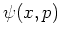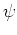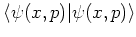Previous: 6.2 Quantum Mechanics in Up: 6.2 Quantum Mechanics in Next: 6.2.2 Definition of Wigner

## 6.2.1 Phase Space Distribution Functions

In 1932 Wigner [Wig32] suggested a representation of quantum mechanics by means of joint distributions of the probabilities (or, more precisely, the quasi-probabilities) for coordinates and momenta in phase space.

The phase space representation only involves concepts that are common to both quantum and classical mechanics. Therefore it is especially convenient in the consistent derivation of quasiclassical methods, in which, for example, one of the interacting systems is described by quantum mechanics and the other by classical theory. We used the phase space representation as a unified formalism for combining quantum (coherent) transport models with classical scattering theories [Shi79].

A number of different phase space distribution functions has been introduced and investigated over the years. Most of them fit into Cohen's class of distribution functions [Coh89]. For the purposes of this thesis, we mainly considered the Wigner distribution function [Tat83], the Kirkwood-Rihaczek distribution [vR60], [PW02], the Husimi function [LSR95], [App00] and the positive P distribution [DGW81]. All these distribution functions are quantum analogons of the classical Liouville function. Practical use (that is, implementation) was only made of the Wigner function.

There is also a complex representation of the single particle Schrödinger equation in phase space [Pru84], [TVF93], [Wlo94], [TVF90]. This is a Schrödinger equation forwith complex. Thenis a positive quantity which is related to the Husimi density. In this complex representation one can then consider, for instance, the hydrodynamical formulation of the Schrödinger equation [dPC97].